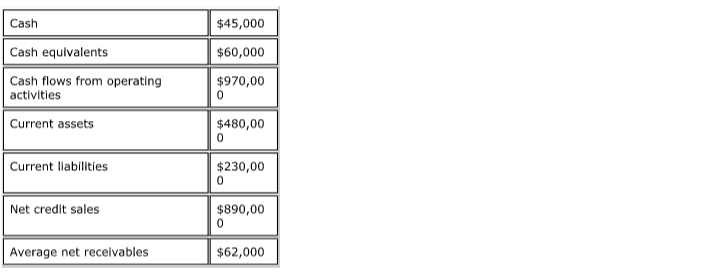# Problem: Consider the following information: Compute the cash ratio to the nearest hundredth. A. 0.46 to 1 B. 0.19 to 1 C. 2.08 to 1 D. 1.73 to 1

###### Problem Details

Consider the following information:

Compute the cash ratio to the nearest hundredth.

A. 0.46 to 1

B. 0.19 to 1

C. 2.08 to 1

D. 1.73 to 1